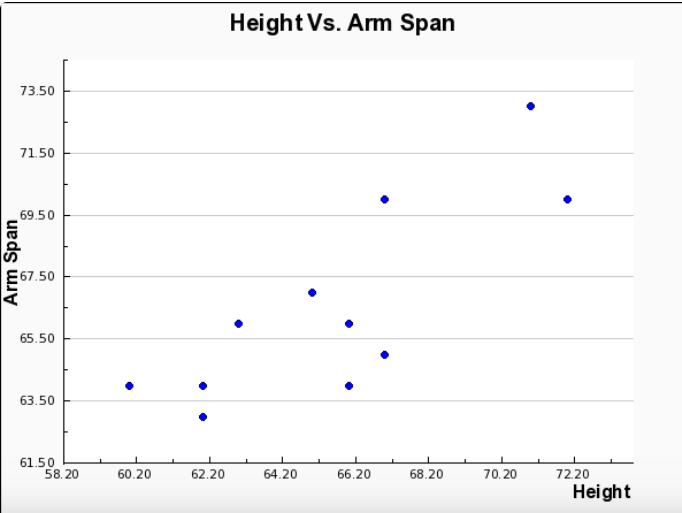James G.

# I did everything else, I just need the rest of part 3

### Part Two: Representation of Data with Plots

1. Using graphing software of your choice, create a scatter plot of your data. Predict the line of best fit, and sketch it on your graph.
2. Copy and paste your scatter plot into a word processing document.

### Part Three: The Line of Best Fit

1. Which variable did you plot on the x-axis, and which variable did you plot on the y-axis? Explain why you assigned the variables in that way.
2. Write the equation of the line of best fit using the slope-intercept formula $y = mx + b$. Show all your work, including the points used to determine the slope and how the equation was determined.
3. What does the slope of the line represent within the context of your graph? What does the y-intercept represent?
4. Test the residuals of two other points to determine how well the line of best fit models the data.
5. Use the line of best fit to help you to describe the data correlation.
6. Using the line of best fit that you found in Part Three, Question 2, approximate how tall is a person whose arm span is 66 inches?
7. According to your line of best fit, what is the arm span of a 74-inch-tall person? 101 inches

Part One: Measurements

Data Record

Height vs. Arm Span

Height (x) in. Arm span ( y) in.Part Two: Representing Data with Plots

Please refer to the attached document for the graphs.

Part Three: The Line of Best Fit

Using excel, the two points that were used to draw the lines of best fits were (62, 63 ) and (70, 72 ).

By:Tutor
5 (12)

Tutor specializing in Economics and Mathematics

## Still looking for help? Get the right answer, fast.

Get a free answer to a quick problem.
Most questions answered within 4 hours.

#### OR

Choose an expert and meet online. No packages or subscriptions, pay only for the time you need.